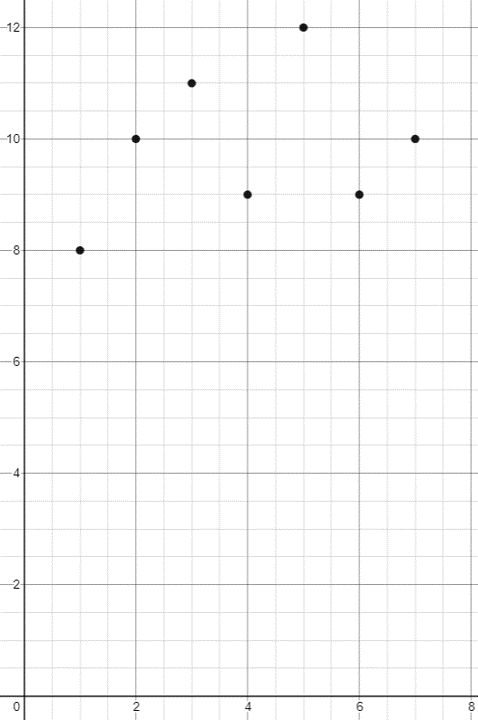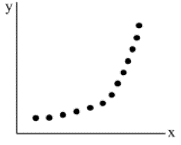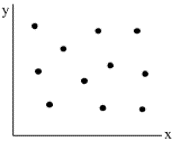# Linear Relationships

A linear relationship is used to describe a straight-line relationship between two variables. It can be expressed as a table of values or a graph where the data points are connected via a straight line. That includes vertical lines and horizontal lines.

Mathematically, a linear relationship is one that satisfies the equation:

$$y = m(x) + b$$

where $$m$$ is the slope and $$b$$ is the y-intercept. Recall the slope formula is the change in y over change in x.

## Table of Values

Every linear equation is a relationship of x and y values. To create a table of values, we just have to pick a set of x values, substitute them into the equation and evaluate to get the y values. You could also read the points from a graph.

Enter the slope and y-intercept below to create a table of values.

## First Difference

The First differences are the differences between consecutive y-values in tables of values with evenly spaced x-values. When the first difference is constant (the same), the relation is linear.

For exmaple, Jameen has recorded his sleep for the week in the table below.

 X(day) Y(hours) Mon Tues Wed Thur Fri Sat Sun 8 10 11 9 12 9 10

To calculate the first difference, we subtract the second y-value form the first:

$$10-8 = 2$$

The difference in the y values are 2,1,-2,3,-3,1. Thus we can determine that the relationship of Jameen's sleep is non-linear.

Use first differences to determine if the table below has a linear or non linear relationship
 X Y 0 1 2 3 4 5 10 13 16 19 22 25

## Scatter Plots

A scatter plot shows the relationship between two variables on a graph. The values of one variable appear on the horizontal axis (independent variable, typically x), and the values of the other variable appear on the vertical axis (dependent variable, typically y).

For exmaple, Jameen has recorded his sleep for the week. The data can also be presented on a graph.

 X(day) Y(hours) Mon Tues Wed Thur Fri Sat Sun 8 10 11 9 12 9 10## Linear vs Non-Linear Relationships

A scatter plot can be used to identify several different types of relationships between two variables.

• The relationship is linear when the points on a scatter plot follow a somewhat straight line pattern.
• The relationship is considered non-linear if the points on a scatterplot follow a pattern but not a straight line
• The relationship has no correlation if the points on the scatterplot do not show any pattern.LinearNon-LinearNo-Correlation

An outlier is a data point that does not follow the general trend.

## Direct vs Indirect Variation

Two variables vary directly (Direct Variation) if they are directly proportional to each other. The relation would have the form $$y = m(x)$$ (i.e., $$b=0$$) and the graph passes through the origin (0,0)..

A partial variation is one where the the value of the dependent variable depends on both the value of the independent variable and some initial value. they are the form $$y = mx + b$$ and the graph never passes through the origin (0,0).

In turn, a variation is inverse if y is expressed as the product of some constant number k and the reciprocal of x, given that k $$\ne$$ 0

Direct Variation Indirect Variation Inverse Variation
$$y=2.5(x)$$ $$y=2.5(x)+4$$ $$y=\cfrac{1}{x}$$
$$y=\cfrac{1}{2}(x)$$ $$y=\cfrac{3}{2}(x)-1/5$$ $$y=\cfrac{-3}{x}$$
$$y=x$$ $$y=x-1$$ $$y=\cfrac{5}{x}$$